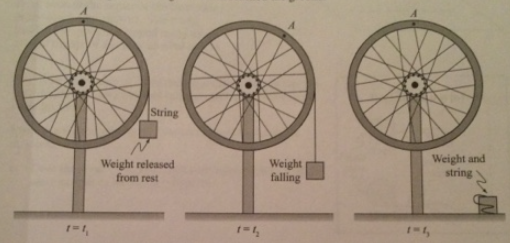# Problem: A Bicycle wheel is mounted on a fixed, frictionless axle. A light string is wound around the wheel's rim, and a weight is attached to the string at its free end. the diagrams below depict the situation at three different instances:At t=t1, the weight is released from rest.At t=t2, the weight is falling and the string is still partially wound around the wheel.At t=t3, the weight and string have both reached the ground.1. What is the direction of the angular velocity of the wheel at the each time shown? If angular velocity is zero at any time, state that explicitly. Explain.2. What is the direction of the wheel's angular acceleration at each time shown? If the angular acceleration is zero at any time, state that explicitly. Explain.3. Rank the magnitudes of the centripetal acceleration of point A at the three times shown. If any of these is zero, state that explicitly. Explain your reasoning

###### FREE Expert Solution

1.

At t = t1, the block has not started falling. This means that there is no torque applied to the wheel.

At t = t1, the angular velocity is zero

At t = t2, we use the right-hand rule.

95% (296 ratings)###### Problem Details

A Bicycle wheel is mounted on a fixed, frictionless axle. A light string is wound around the wheel's rim, and a weight is attached to the string at its free end. the diagrams below depict the situation at three different instances:
At t=t1, the weight is released from rest.
At t=t2, the weight is falling and the string is still partially wound around the wheel.
At t=t3, the weight and string have both reached the ground.1. What is the direction of the angular velocity of the wheel at the each time shown? If angular velocity is zero at any time, state that explicitly. Explain.
2. What is the direction of the wheel's angular acceleration at each time shown? If the angular acceleration is zero at any time, state that explicitly. Explain.
3. Rank the magnitudes of the centripetal acceleration of point A at the three times shown. If any of these is zero, state that explicitly. Explain your reasoning